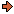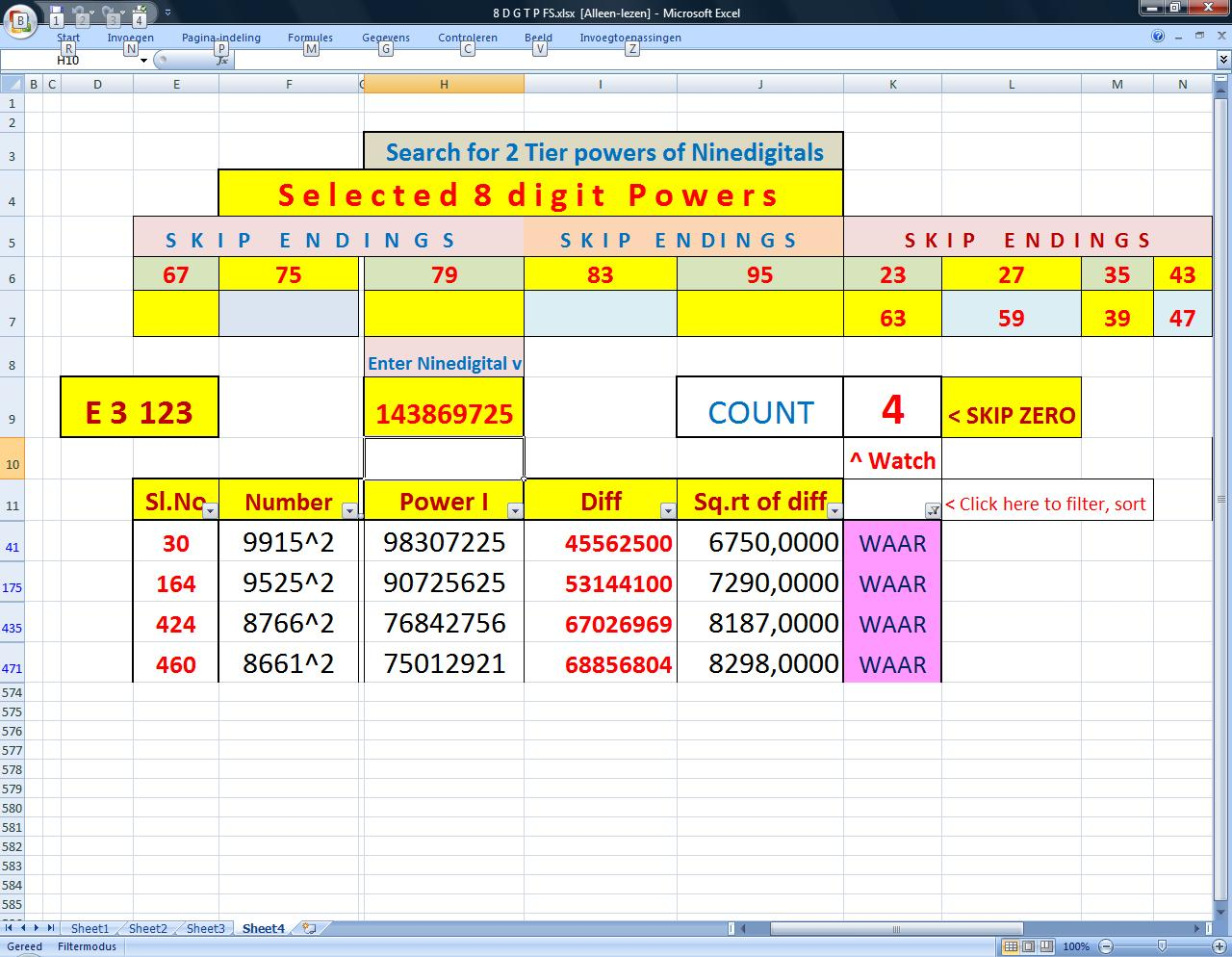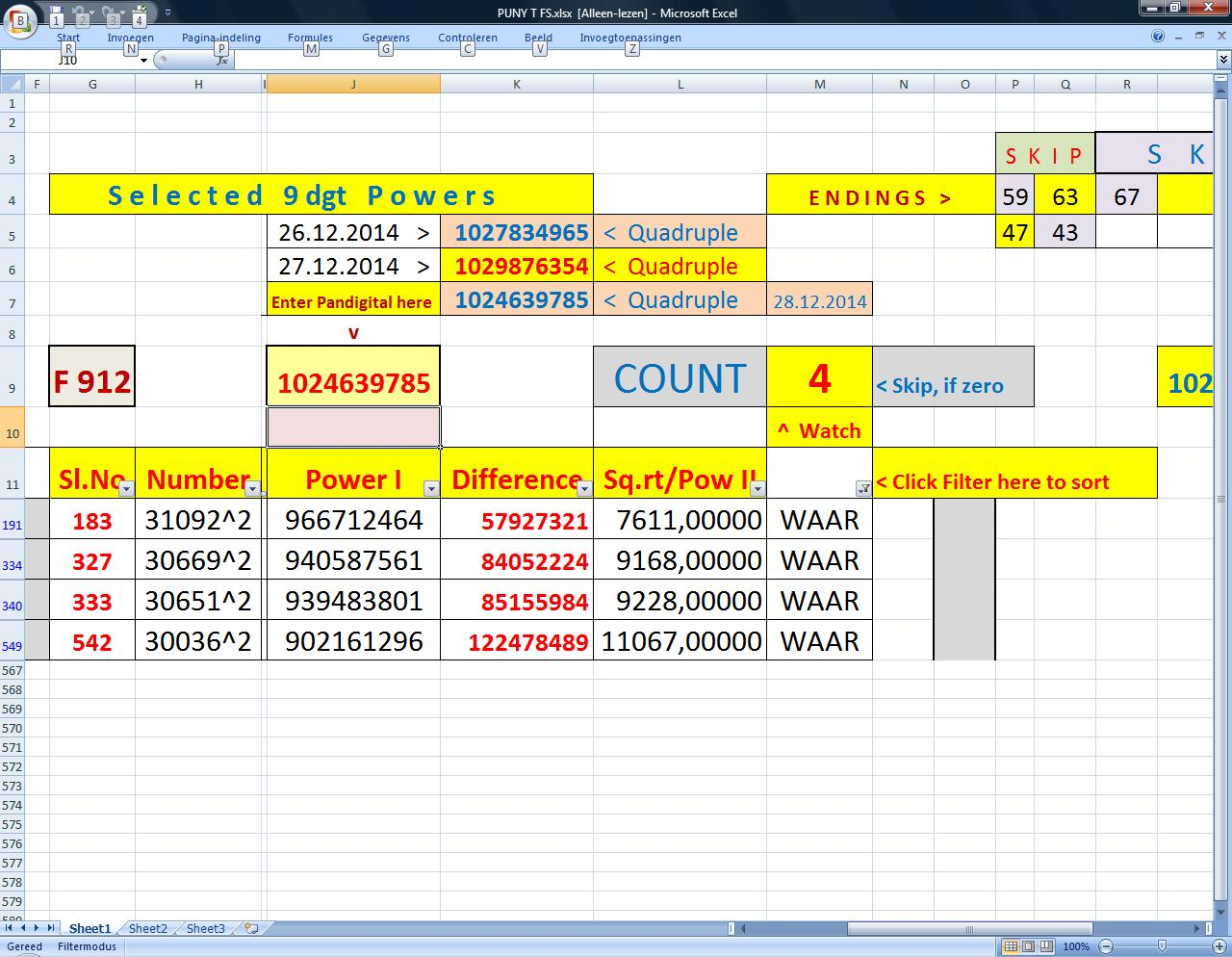World!Of
NumbersWON plate
193 |

[ February 1, 2015 ]
Exploring nine- and pandigitals
expressible as sums of two squares
B.S. Rangaswamy (email)

B.S. Rangaswamy enclosed me 2_tier constituents of powers for ninedigitals and pandigitals.
Some have double, triple, quadruple, quintuple and even a sevenfold set of constituents.
He used clever Excel table programming to get some selected (restricted) results
where one of the two powers is an eight-digit number in the case of ninedigitals
and a nine-digit number in the case of pandigitals (the 2nd powers are only resultants).
The filtering and sorting was speeded up with the help of his grandson Puneeth
and daughter in law Indira Ramesh.
Alas, I cannot reproduce the programs in HTML, so I will stick to
displaying partly results and some magical curios for some chosen categories
but without the restrictions imposed by B.S. Rangaswamy.
Interested and involving readers may try to discover existence of other
strange constituents for any of the remaining vast number of nine- and/or pandigitals.

Ninedigital highlights

 Here are already some curios (multi solutions per ninedigital allowed) 143625897 = 98762 + 67892   Note the reversals ! 143752968 = 84782 + 84782   Twice the same term ! 145897362 = 85412 + 85412   Here also twice the same term ! 162973458 = 90272 + 90272   And again twice the same term ! 143689725 = 88502 + 80852   Both terms are anagrams of each other ! 145973682 = 98612 + 69812   Bis - both terms are anagrams of each other ! 149683725 = 87542 + 85472   Ter - both terms are anagrams of each other !

Statistical information and more curios are to be found
in one of my dedicated ninedigits pages. Please visit alsoStatistics and curios
 You noticed already that all values A and B are multiples of 3. How come ?This due to the fact that every ninedigital is divisible by 9 (all digits sums to 45). In order to be able to separate the 9-factor from the sum of squares, each value A and B must be a multiple of 3. Idem dito for the pandigitals. N = A2 + B2 9 * N' = 9 * ( A'2 + B'2 ) 9 * N' = ( 9 * A'2 ) + ( 9 * B'2 ) 9 * N' = ( 3 * A' )2 + ( 3 * B' )2

Case N.1ninedigital = strictly single solutions for A2 + B2

 A and B restricted to 4-digit numbers
 123456978 = 52232 + 98072 [ Smallest ] 123458976 = 74762 + 82202 Many more solutions exist [ Solutions must be < 200000000 ] 193675842 = 97292 + 99512 194265378 = 97472 + 99632 [ Largest ]

 A and B restricted to 5-digit numbers
 213576498 = 101372 + 105272 312457986 = 120692 + 129152 412536978 = 127472 + 158132 512374986 = 138152 + 179312 612345978 = 108032 + 222632 712345896 = 142142 + 225902 812356794 = 193652 + 209132 912346857 = 131012 + 272162 [ A selection of solutions ]

Case N.2ninedigital = strictly double solutions for A2 + B2

 A and B restricted to 4-digit numbers

Solutions must be < 200000000.

 123475986 = 68252 + 87692 = 69692 + 86552 152367489 = 95672 + 78002 = 95402 + 78332 [ Many more solutions exist ]

 A and B restricted to 5-digit numbers
 245193768 = 100382 + 120182 = 108422 + 112982 312685749 = 106952 + 140822 = 113102 + 135932 412365978 = 111332 + 169832 = 140132 + 146972 512387493 = 136862 + 180302 = 145862 + 173102 [ Many more solutions exist ]

Case N.3ninedigital = strictly triple solutions for A2 + B2
Note that these triples are sparingly scattered.

 A and B restricted to 4-digit numbers

 135697428 = 64022 + 97322 = 68282 + 94382 = 80522 + 84182 [ a strictly _unique_ triple solution ]

 A and B restricted to 5-digit numbers
 326987541 = 104552 + 147542 = 119462 + 135752 = 122462 + 133052 [ Smallest ]

More solutions exist.

Case N.4ninedigital = quadruple solutions for A2 + B2

 A and B restricted to 4-digit numbers
No luck, there are no strictly quadruple solutions for A2 + B2

The next best thing is to search for them as a subset in higher x-fold solutions,
as for instance in the following 12-fold example.
 143869725 = 99152 + 67502 = 95252 + 72902 = 87662 + 81872 = 86612 + 82982 [ eight more when unrestricted ]

 A and B restricted to 5-digit numbers
 415869273 = 110372 + 171482 = 121922 + 163472 = 132722 + 154832 = 143072 + 145322 [ smallest ]

More solutions exist.

Case N.5ninedigital = quintuple solutions for A2 + B2

 A and B restricted to 4-digit numbers
There exist no strictly quintuple solutions for A2 + B2

The next best thing is to search for them as a subset in higher x-fold solutions,
as for instance in the following 12-fold example from B.S. Rangaswamy.
 143697825 = 97772 + 69362 = 97322 + 69992 = 94442 + 73832 = 92762 + 75932 = 89132 + 80162 [ seven more when unrestricted ]

 A and B restricted to 5-digit numbers
I haven't encountered a strictly quintuple solution of this kind. It doesn't exist.

The next best thing is to search for them as a subset in higher x-fold solutions,
as for instance in the following 18-fold example.
 346978125 = 101102 + 156452 = 110852 + 149702 = 121622 + 141092 = 126752 + 136502 = 131462 + 131972 [ smallest ][ 13 more when unrestricted ]

Case N.6ninedigital = sextuple solutions for A2 + B2

 A and B restricted to 4-digit numbers
No luck, there are no strictly sextuple solutions for A2 + B2

The next best thing is to search for them as a subset in higher x-fold solutions,
but alas, here also there are NO solutions.

 A and B restricted to 5-digit numbers
No luck either, there are no strictly sextuple solutions for A2 + B2

The next best thing is to search for them as a subset in higher x-fold solutions,
as for instance in the following 12-fold example.
 419867325 = 100142 + 178772 = 109652 + 173102 = 121622 + 164912 = 124262 + 162932 = 130352 + 158102 = 143582 + 146192 [ smallest ][ six more when unrestricted ] Other subset sextuple solutions are 469371825, 469738125, 487392165, 569384712, 597632841, 618394725, 631849725, 634189725, 637184925, 643198725, 679238145, 679431285, 679843125, 684793125, 684925137, 689173425, 691437825, 692731845, 697354281, 698317425, 718643925, 724961385, 736841925, 741268593, 761943825, 764318925, 764893125, 769413285, 769438125, 781936425, 783952416, 784319562, 793461825, 794318625, 813764925, 813796425, 817923465, 819347625, 825641973, 841796325, 845629317, 847693125, 861473925, 864173925, 874169325, 876941325, 893647521, 893765412, 894132765, 894673125, 895734216, 896473125, 912374865, 918376425, 925764138, 926457381, 932147658, 932765418, 934768125, 936784125, 938671425, 942716385, 943218765, 946783125, 947138625, 964873125, 967354128, 967843125, 971438625, 974683125, 976412385, 976823145, 981324765, 986371425 and 987342165

Case N.7ninedigital = sevenfold solutions for A2 + B2

 A and B restricted to 4-digit numbers
There exist no strictly 7-fold solutions for A2 + B2

The next best thing is to search for them as a subset in higher x-fold solutions,
as for instance in the following 24-fold example from B.S.Rangaswamy.
 139876425 = 98762 + 65072 = 96992 + 67682 = 96122 + 68912 = 92132 + 74162 = 90452 + 76202 = 90122 + 76592 = 84452 + 82802 [ 17 more when unrestricted ]

But the first occurence happens within the following 16-fold ninedigital
 124879365 = 54392 + 97622 = 56492 + 96422 = 60542 + 93932 = 63422 + 92012 = 65462 + 90572 = 71672 + 85742 = 73772 + 83942 [ smallest ][ nine more when unrestricted ]

 A and B restricted to 5-digit numbers
There exist no strictly 7-fold solutions for A2 + B2

The next best thing is to search for them as a subset in higher x-fold solutions,
as for instance in the following 8-fold example.
 627498153 = 112772 + 223682 = 127322 + 215732 = 132032 + 212882 = 154832 + 196922 = 158132 + 194282 = 159122 + 193472 = 171572 + 182522 [ one more when unrestricted ] Other subset sevenfold solutions are 631984725, 681257394, 683791425, 719486325, 734891625, 738469125, 738694125, 745631289, 746193825, 748931625, 764391825, 781249365, 781634925, 789245613, 791648325, 792813645, 813469725, 817364925, 831476925, 832176945, 841396725, 846139725, 846912573, 849273165, 851327649, 861734925, 861934725, 864379125, 864713925, 867495213, 867913425, 876931425, 879361425, 893417265, 896714325, 914732685, 914863725, 916873425, 917483265, 918476325, 924163578, 938647125, 946731825, 961438725, 964378125, 978146325, 982675413, 983167425, 984127365 and 986731425

Case N.8ninedigital = eightfold solutions for A2 + B2

 A and B restricted to 5-digit numbers

 895723146 = 120152 + 274112 = 157892 + 254252 = 167612 + 247952 = 172052 + 244892 = 177392 + 241052 = 201152 + 221612 = 205112 + 217952 = 209852 + 213392 [ a strictly _unique_ eightfold solution ] Other subset eightfold solutions are 463879125, 647193825, 691847325, 719348625, 761438925, 763849125, 789346125, 794386125, 794631825, 817639425, 817693425, 821749365, 831249765, 846731925, 879461325, 879463125, 971382465 and982715634

Case N.9ninedigital = higher-fold solutions for A2 + B2

 A and B restricted to 5-digit numbers

 9-fold solutions 728931645 subset from a 16-fold 734189625 subset from a 16-fold 739241685 subset from a 16-fold 837612945 subset from a 16-fold 873469125 subset from a 16-fold 893127645 subset from a 16-fold 897134625 subset from a 16-fold 912634785 subset from a 16-fold 914273685 subset from a 16-fold 948376125 subset from a 16-fold 963417285 subset from a 16-fold 971436258 subset from a 16-fold 981342765 subset from a 16-fold 10-fold solutions 417938625 subset from a 24-fold 783961425 subset from a 18-fold 987634125 subset from a 16-fold 11-fold solutions 439817625 subset from a 32-fold 763498125 subset from a 20-fold 796843125 subset from a 20-fold 946837125 subset from a 16-fold 961342785 subset from a 16-fold 971238645 subset from a 16-fold 973864125 subset from a 16-fold 12-fold solutions 698341725 subset from a 24-fold 726193845 subset from a 24-fold 968417325 subset from a 18-fold 13-fold solutions 839147625 subset from a 24-fold 913276845 subset from a 24-fold 14-fold solutions 869371425 subset from a 24-fold 15-fold solutions 943678125 subset from a 24-fold

 Why is it that some ninedigitals have more 'sum of squares' solutions than others?This is due to the fact every ninedigital has its unique factorization. Take for instance 439817625 which has nine small prime factors. 3 * 3 * 5 * 5 * 5 * 13 * 17 * 29 * 61 This allows for many combinations and thus eligible solutions whereas e.g. 123458679 = 3 * 3 * 13717631 has only three prime factors and hence impossible to express as a sum of squares.

Case N.N.1Lowest and highest ninedigitals for A2 + B2

 A and B restricted to 4-digit numbers

 123456978 = 52232 + 98072   is the lowest ( from a strictly single solution ) 194265378 = 97472 + 99632   is the highest ( in case of a strictly single solution ) 198463725 = 99422 + 99812   is one highest version from a multi_3 solution

 A and B restricted to 5-digit numbers

 213458697 = 100592 + 105962   is the lowest ( ie. the highest version from a multi_2 solution ) 213576498 = 101372 + 105272   is the lowest ( in case of a strictly single solution ) 987641253 = 118232 + 291182   is the highest ( in case of a strictly single solution ) 987654321 = 182642 + 255752   is one highest version from a multi_3 solution

Case N.N.2Apart from squares there are cubes & other powers
as 2_tier constituents of ninedigitals
but that will be a topic for a separate investigation in the future.

 125734689 = 4503 + 58832 136725489 = 84332 + 904 137865924 = 4323 + 75662 137965248 = 4473 + 69752 138267594 = 4173 + 81092 138427956 = 4353 + 74912 138629457 = 88412 + 610 139487652 = 964 + 73862 139842756 = 964 + 74102 139854276 = 89102 + 610 142687953 = 4383 + 76592

Case N.N.3ninedigital = single solutions for A3 + B2

125734689 = 4503 + 58832
145273689 = 4623 + 68312

Case N.11ninedigital = single solutions for A4 + B2

123497685 = 934 + 69782
123498756 = 964 + 62102B.S. Rangaswamy wishes all of us a happy new year 2015 ( = 113 + 262 + 23)

Pandigital highlights

Case P.1pandigital = single solutions for A2 + B2
( A and B not restricted ).

Lots of solutions are known...

 1029846537 = 300962 + 111392 2345679018 = 292832 + 385772 6098752314 = 377672 + 683552

Case P.2pandigital = double solutions for A2 + B2
( A and B not restricted ).

Many more solutions exist...

 1023457698 = 315332 + 53972 = 303632 + 100772 1029853476 = 315302 + 59762 = 308402 + 88742 9712304685 = 315542 + 933632 = 557582 + 812612

Case P.3pandigital = triple solutions for A2 + B2.

 A and B restricted to 5-digit numbers

 1034962857 = 102212 + 305042 = 160592 + 278762 = 212612 + 241442

Case P.4pandigital = quadruple solutions for A2 + B2
( A and B not restricted ).

 1023698754 = 317732 + 37652 = 315152 + 55232 = 312152 + 70232 = 307772 + 87452 1083456729 = 310052 + 110522 = 306272 + 120602 = 304202 + 125732 = 294752 + 146522

Case P.5pandigital = quintuple solutions for A2 + B2

Who can provide me with the first example ?

Case P.6pandigital = sextuple solutions for A2 + B2

Who can provide me with the first example ?

Case P.7pandigital = sevenfold solutions for A2 + B2

Who can provide me with the first example ?

Case P.P.1Lowest and highest pandigital for A2 + B2
( A and B not restricted ).

 1023456789 = 313832 + 62102 is the lowest version 1 1023456789 = 293672 + 126902 is the lowest version 2 9876435210 = 306032 + 945512 is one of eight highest versions

Case P.P.2Already one nice curio combining two numbertypes
(pandigital) 1024397685 = 314822 + 57692 (ninedigital)

Case P.P.3More nice curios in case of higher exponents
 1052736489 = 9923 + 87492   Both basenumbers are indivisible by either 3 or 9 8197604352 = 20162 + 20163   See you next year !

Case P.P.4ninedigital = double solutions for A3 + B2
 1023597648 = 9993 + 51572 = 9723 + 102602Database

Note: this list is very lengthy !
Creating a complete list might be unrealistic, so I will replace it
with a UBASIC program someday.

```

```A000193Prime Curios!Prime PuzzleWikipedia 193Le nombre 193```

```

[TOP OF PAGE]

Patrick De Geest - Belgium- Short Bio - Some Pictures